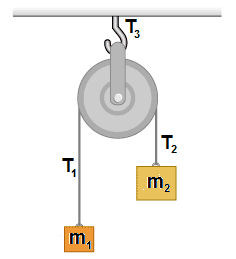# Finding the tension in a pulley that rotates

PhyIsOhSoHard

## Homework Statement$m_2>m_1$
The pulley rotates.
Find the tensions, $T_1, T_2, T_3$

Newton's laws

## The Attempt at a Solution

I know how to solve the problem if the pulley doesn't rotate because the systems would be in equilibrium (Newton's first law) and dividing into 3 systems (one for each tension) you get $T_1=m_1g$, $T_2=m_2g$ and $T_3=m_1g+m_2g$.

But I have no idea what changes when the pulley starts rotating. Can somebody give me some leads?